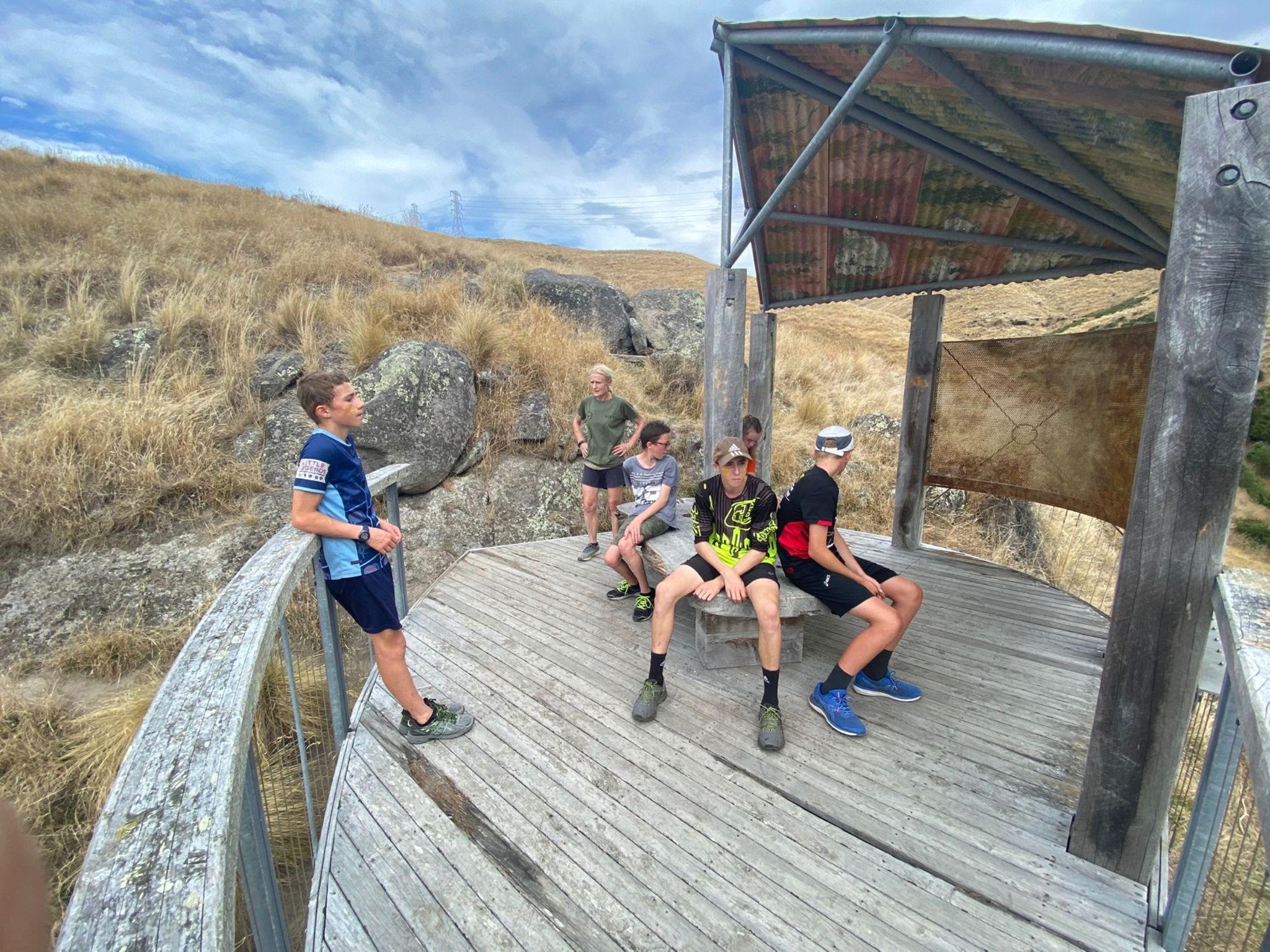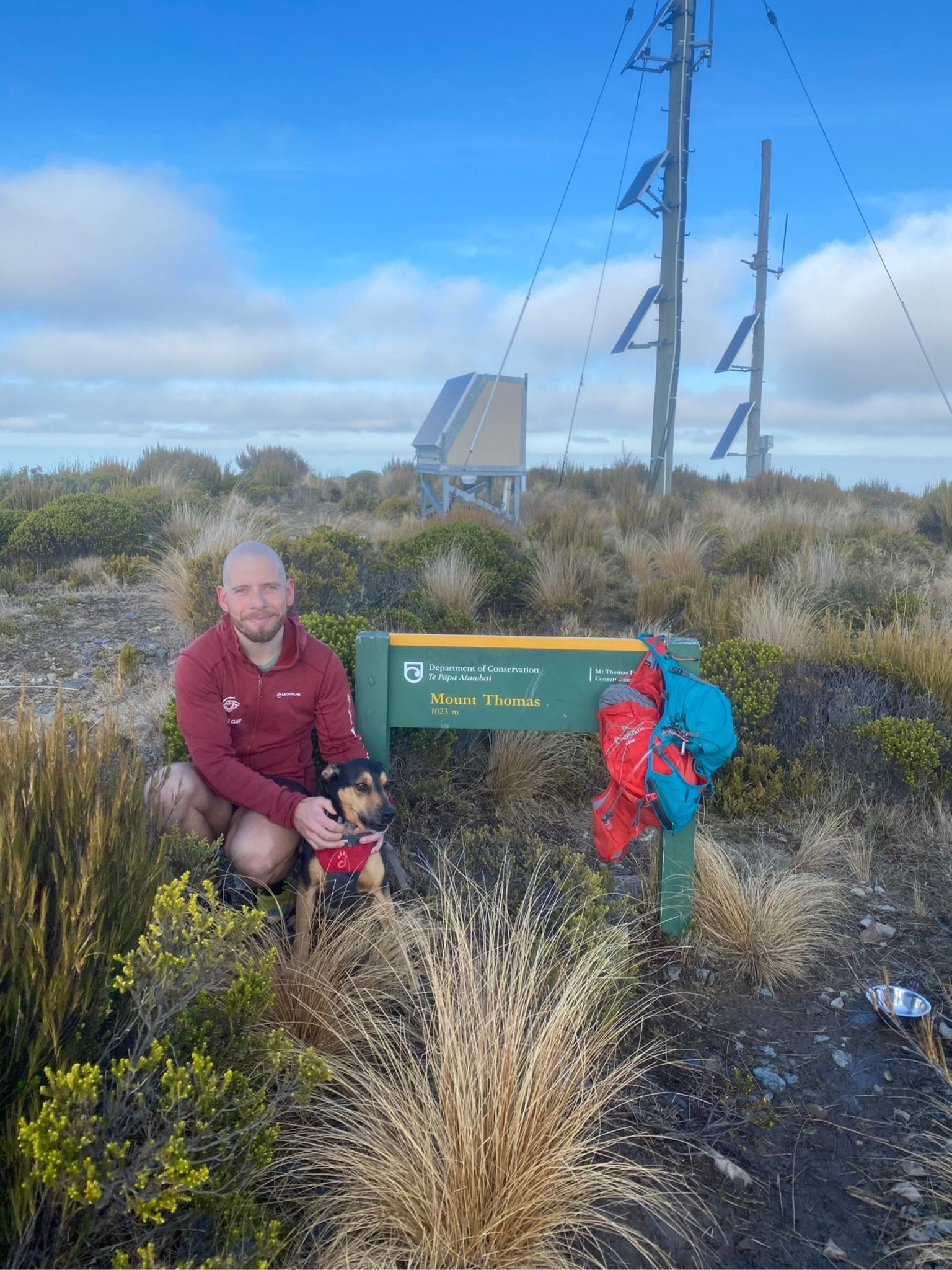Skip to main content

## Ngā Whāinga Ako

1. Name the metric units of distance, time and speed and give their symbols.
2. Define the term speed.
3. Calculate the average speed of objects. Use $v=d/t$.

## What is Speed?

You know that someone who finishes a race in the shortest time is the fastest. That they have the highest speed.

Speed is the amount of distance covered in some amount of time.

## What is Speed?

• Cars display their speed in kilometers per hour
• In Physics we prefer to give speed in the number of meters travelled in one second.
• We call this meters per second.## Calculating Speed

In order to determine speed we need to know two things!

• Distance: has unit meters (m) and is represented by symbol $d$ in equations.
• Time: has unit seconds (s) and is represented by symbol $t$ in equations.

## Speed vs Velocity

• Speed is a measure of distance covered in a certain amount of time.
• e.g 13 meters per second
• Velocity includes a direction
• e.g. 13 meters per second east

## How to Calculate Velocity

\begin{aligned} velocity &= \frac{\text{change in distance}}{\text{change in time}} \\ v &= \frac{\Delta d}{\Delta t} \\ \end{aligned}

It is measured in meters per second, also shown as $\frac{m}{s}$ or $ms^{-1}$.

1. Knowns
2. Unknowns
3. Formula
4. Substitute
5. Solve

## Question 1: Velocity

A rock climber climbs 15 meters in 5.48 seconds. What velocity was he travelling?## Question 1: Answer

1. Knowns: $\text{distance (d)}=15m$, $\text{time (t)} = 5.48s$
2. Unknowns: $\text{velocity (v)}$
3. Formula: $v = \frac{\Delta d}{\Delta t}$
4. Substitute: $v = \frac{15}{5.48}$
5. Solve: $v = 2.74\frac{m}{s}$

## Question 2

• Mr Le Sueur ran 4.61km in 21 minutes and 16 seconds. How fast did he run?
• Hint: Convert the time and distance to Standard International Units## Question 2: Answer

1. Knowns: $\text{distance (d)} = 4.61km = 4610m$, $\text{time (t)} = 21min, 16s = 1276s$
2. Unknowns: $\text{velocity (v)}$
3. Formula: $v = \frac{\Delta d}{\Delta t}$
4. Substitute: $v = \frac{4610}{1276}$
5. Solve: $v = 3.61\frac{m}{s}$

## Question 3• Mr Le Sueur ran at $1.51\frac{m}{s}$ for 1hr, 18min and 11 seconds. How far did he run?
• Hint: Convert the time to Standard International Units

## Question 3: Answer

1. Knowns: $\text{velocity (v)} = 1.51\frac{m}{s}$, $\text{time (t)} = 1hr, 18min, 11s = 4691s$
2. Unknowns: $\text{distance (d)}$
3. Formula: $v = \frac{\Delta d}{\Delta t}$
4. Substitute: $1.51 = \frac{d}{4691}$
5. Solve: $1.51 \times 4691 = d = 7083.41m$

## Mahi Kāinga

Due Monday, August 24th: Mahi Kāinga Booklet Q2, Q3, Q1